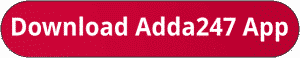Latest Banking jobs   »   Quantitative Aptitude Quiz For SBI PO/Clerk...

# Quantitative Aptitude Quiz For SBI PO/Clerk Prelims 2023 -30th October

Directions (1-5): In each of these questions, two equation (I) and (II) are given. You have to solve both the equations and give answer
(a) If x>y
(b) If x≥y
(c) If x<y
(d) If x≤y
(e) If x = y or no relation can be established between x and y

Q1. I. x²-196=0
II. y=√196

Q2. I. x²-2x-15=0
II. y²-7y-18=0

Q3. I. x²-x-30=0
II. y²+16y+64=0

Q4. I. 2x²-17x+36=0
II. y²+4y-21=0

Q5. I. 2x²-15x+28=0
II. 2y²+17y+35=0

Directions (6-10): In each of these questions, two equation (I) and (II) are given. You have to solve both the equations and give answer
(a) If x>y
(b) If x≥y
(c) If x<y
(d) If x≤y
(e) If x = y or no relation can be established between x and y

Q6. I. x³=512
II. y²+10y+16=0

Q7. I. x²-17x+72=0
II. y²-81=0

Q8. I. 2x²-29x-48=0
II. 3y²-y-30=0

Q9. I. x²+7x-60=0
II. y²-14y+45=0

Q10. I. x²-x-56=0
II. y²-12y+32=0

Solutions.                                                           .                                                          .                                                     .

.                                                           .                                                          .                                                     .

.                                                           .                                                          .                                                     .

## FAQs

### What sections are included in the SBI PO or SBI Clerk Prelims Exam?

The selection process of SBI Clerk 2023 consists of Prelims, Mains and LPT.

#### Congratulations!Union Budget 2023-24: Free PDF